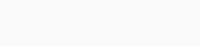# Matlab R2019b v9.7.0 Update 4 (windows / macos)

### Matlab R2019b

Matlab is a software that provides a numerical computing environment and is a high-level programming language. Using Matlab you can calculate and solve some technical problems with matrices, plot information graphs or graph functions. Implementing algorithms, creating user interfaces, it is also capable of combining with computer programs written in many other programming languages.

### Key Features in Matlab R 2019

• Development environment for managing code, files, and data.
• Perform complex mathematical calculation methods.
• Explore helps you discover ways to get to a solution faster.
• It has a wide range of mathematical functions for linear algebra, optimization, filtering, statistics, Fourier analysis, numerical integration and many more…
• You can draw two-dimensional or three-dimensional graphical functions to visualize the information to be presented.
• Design and build user interfaces using C++, C or Java programming languages.
• There are many features and tools from engineering companies for specific applications such as control, fuzzy, telecommunications, estimation, neural networks, probability,  , statistics, data collection, simulation system,…
• Computational biology.
• It is also capable of testing and measuring functions and charts accurately.
• In addition, it is capable of handling media signals, images and videos.